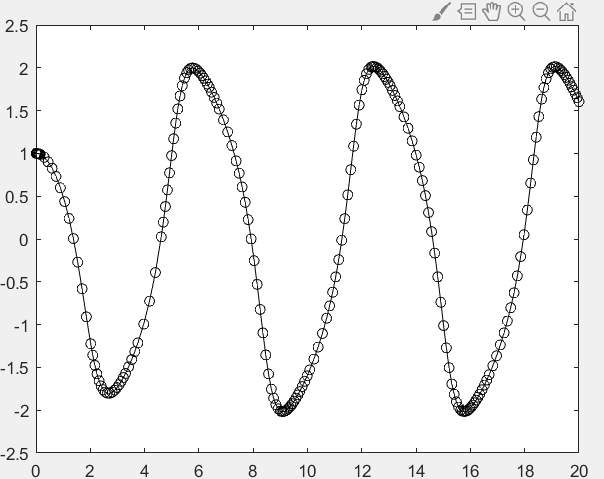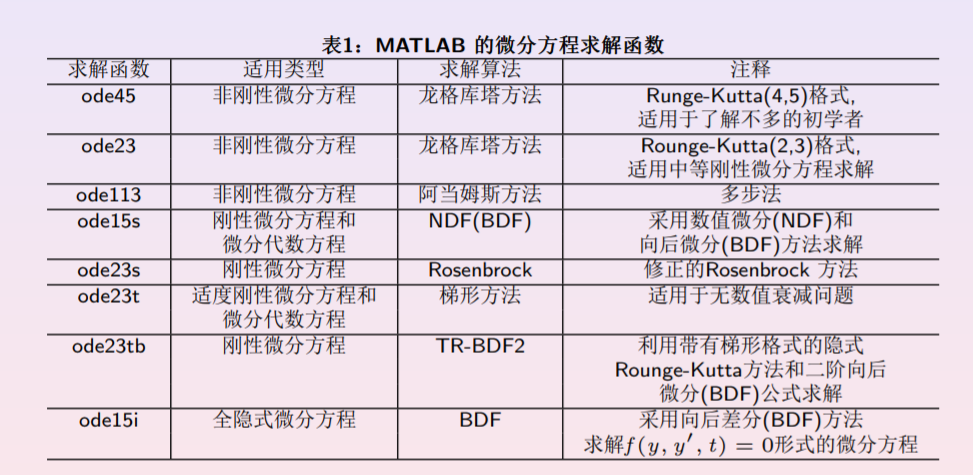# matlab中常微分方程数值计算的方法

## 欧拉格式：

向后欧拉格式，也叫作向前欧拉格式

## matlab函数

dsolve('方程式',‘条件’,'变量')

y=dsolve('D3y-D2y=x','y(1)=8','Dy(1)=7','Dy(2)=4','x')

求数值解，用龙格-库塔方法            [t,y]=ode45(odefun,tspan,y0)

odefun 为 以表示微分方程的右端项 的函数句柄或者 内联函数

tspan 表示自变量的范围

y0 是微分方程的初始值向量

f=@(x,y)-2*y+2*x^2+2*x;
[x,y]=ode45(f,[0,0.5],1)

[t,Y]=ode45(@vdp,[0 20],[1 0]);
plot(t,Y(:,1),'k-o');

function dy= vdp(t,y)
dy=[y(2);(1-y(1)^2)*y(2)-y(1)];
end06-24381

#### matlab学习笔记第七章——常微分方程(ODE)的数值解04-305814

#### 常微分方程的解法 (四): Matlab 解法

04-138003

#### 常微分方程的数值求解

01-275527

#### 数值分析 第七章 常微分方程的数值解法

12-294167

#### 常微分方程数值解法——python实现

06-1298

#### MATLAB-6-5常微分方程数值求解

07-085万+

#### Matlab(1)使用ode45求解常微分方程

05-202700

#### 常微分方程matlab求解©️2020 CSDN 皮肤主题: 大白 设计师: CSDN官方博客点击重新获取扫码支付1.余额是钱包充值的虚拟货币，按照1:1的比例进行支付金额的抵扣。
2.余额无法直接购买下载，可以购买VIP、C币套餐、付费专栏及课程。余额充值